Courses

WBJEE Subjective Mathematics Sample Paper II Notes | Study WBJEE Sample Papers, Section Wise & Full Mock Tests - JEE

JEE: WBJEE Subjective Mathematics Sample Paper II Notes | Study WBJEE Sample Papers, Section Wise & Full Mock Tests - JEE

The document WBJEE Subjective Mathematics Sample Paper II Notes | Study WBJEE Sample Papers, Section Wise & Full Mock Tests - JEE is a part of the JEE Course WBJEE Sample Papers, Section Wise & Full Mock Tests.
All you need of JEE at this link: JEE

Question: 1. Prove that the equation cos2x + a sinx = 2a – 7 possesses a solution if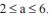Ans: ⇒ cos2x + a sinx = 2a – 7
⇒ 2sin2x – asinx + (2a – 8) = 0
Since sin ∈IR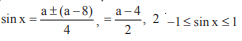∴ Given equation has solution ofQuestion: 2. Find the values of x, (–S < x < S, x ≠ 0) satisfying the equation,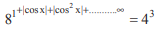Ans: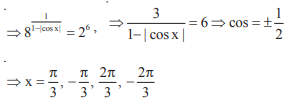Question: 3.  Prove that the centre of the smallest circle passing through origin and whose centre lies on y = x + 1 is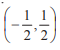Ans:
Let centre be c(h, h + 1) , 0(0, 0)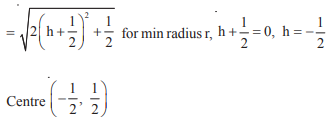Question: 4. Prove by induction that for all n∈ N, n2 + n is an even integer (n ≥ 1)
Ans:  x = 1, x2 + x = 2 is an even integer
Let for n = k, k2 + k is even
Now for n = k + 1, (k + 1)2 + (k + 1) – (k2 + k)
= k2 + 2k + 1 + k + 1 – k2 – k = 2k + 2 which is even integer also k2 + k is even integer
Hence (k + 1)2 + (k + 1) is also an even integer
Hence n2 + n is even integer for all n∈ N.

Question: 5. If A, B are two square matrices such that AB = A and BA = B, then prove that B2 = B
Ans:  B2 = B.B = (BA)B = B (AB) = B(A) = BA = B (Proved)

Question: 6.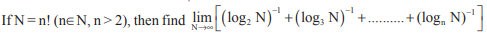Ans: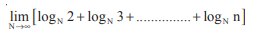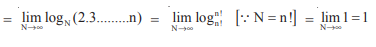Question: 7. Use the formula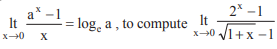Ans: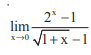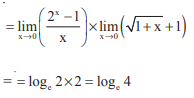Question: 8.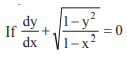prove that,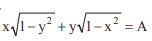where A is constant
Ans: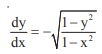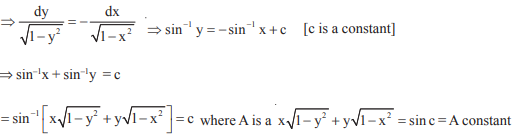Question: 9. Evaluate the following integral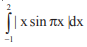Ans: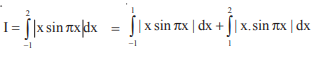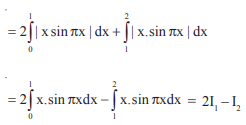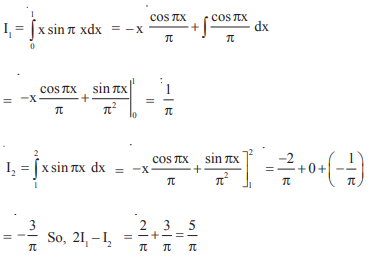Question: 10.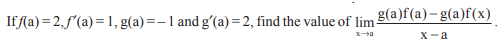Ans: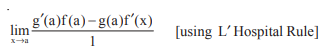= g'(a) f(a) – g(a) f'(a)
= (2)(2) – (–1) (1) = 4 + 1 = 5

The document WBJEE Subjective Mathematics Sample Paper II Notes | Study WBJEE Sample Papers, Section Wise & Full Mock Tests - JEE is a part of the JEE Course WBJEE Sample Papers, Section Wise & Full Mock Tests.
All you need of JEE at this link: JEEUse Code STAYHOME200 and get INR 200 additional OFF Use Coupon Code

Top Courses for JEEWBJEE Sample Papers, Section Wise & Full Mock Tests

4 videos|10 docs|54 tests

Top Courses for JEETrack your progress, build streaks, highlight & save important lessons and more!

,

,

,

,

,

,

,

,

,

,

,

,

,

,

,

,

,

,

,

,

,

,

,

,

;